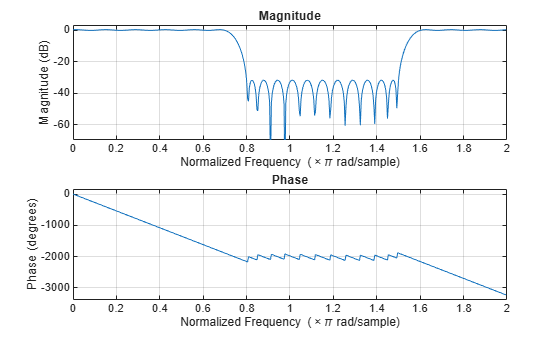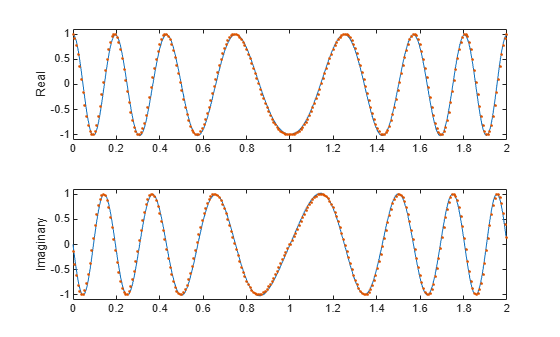# cfirpm

Complex and nonlinear-phase equiripple FIR filter design

## Syntax

```b = cfirpm(n,f,@fresp) b = cfirpm(n,f,@fresp,w) b = cfirpm(n,f,a) b = cfirpm(n,f,a,w) b = cfirpm(...,'sym') b = cfirpm(...,'skip_stage2') b = cfirpm(...,'debug') b = cfirpm(...,{lgrid}) [b,delta] = cfirpm(...) [b,delta,opt] = cfirpm(...) ```

## Description

`cfirpm` allows arbitrary frequency-domain constraints to be specified for the design of a possibly complex FIR filter. The Chebyshev (or minimax) filter error is optimized, producing equiripple FIR filter designs.

`b = cfirpm(n,f,@fresp)` returns a length `n+1` FIR filter with the best approximation to the desired frequency response as returned by function `fresp`, which is called by its function handle (`@fresp`). `f` is a vector of frequency band edge pairs, specified in the range -1 and 1, where 1 corresponds to the normalized Nyquist frequency. The frequencies must be in increasing order, and `f` must have even length. The frequency bands span `f(k)` to `f(k+1)` for `k` odd; the intervals `f(k+1)` to `f(k+2)` for `k` odd are “transition bands” or “don't care” regions during optimization.

Predefined `fresp` frequency response functions are included for a number of common filter designs, as described below. (See Create Function Handle for more information on how to create a custom `fresp` function.) For all of the predefined frequency response functions, the symmetry option `'sym'` defaults to `'even'` if no negative frequencies are contained in `f` and `d` = `0`; otherwise `'sym'` defaults to `'none'`. (See the `'sym'` option below for details.) For all of the predefined frequency response functions, `d` specifies a group-delay offset such that the filter response has a group delay of `n/2+d` in units of the sample interval. Negative values create less delay; positive values create more delay. By default `d` = `0`:

• `@lowpass`, `@highpass`, `@allpass`, `@bandpass`, `@bandstop`

These functions share a common syntax, exemplified below by `@lowpass`.

`b = cfirpm(n,f,@lowpass,...)` and

`b = cfirpm(n,f,{@lowpass,d},...)` design a linear-phase (`n/2+d` delay) filter.

Note

For `@bandpass` filters, the first element in the frequency vector must be less than or equal to zero and the last element must be greater than or equal to zero.

• `@multiband` designs a linear-phase frequency response filter with arbitrary band amplitudes.

`b = cfirpm(n,f,{@multiband,a},...)` and

`b = cfirpm(n,f,{@multiband,a,d},...)` specify vector `a` containing the desired amplitudes at the band edges in `f`. The desired amplitude at frequencies between pairs of points `f(k)` and `f(k+1)` for `k` odd is the line segment connecting the points `(f(k),a(k))` and `(f(k+1),a(k+1))`.

• `@differentiator` designs a linear-phase differentiator. For these designs, zero-frequency must be in a transition band, and band weighting is set to be inversely proportional to frequency.

`b = cfirpm(n,f,{@differentiator,fs},...)` and

`b = cfirpm(n,f,{@differentiator,fs,d},...)` specify the sample rate `fs` used to determine the slope of the differentiator response. If omitted, `fs` defaults to 1.

• `@hilbfilt` designs a linear-phase Hilbert transform filter response. For Hilbert designs, zero-frequency must be in a transition band.

`b = cfirpm(n,f,@hilbfilt,...)` and

`b = cfirpm(N,F,{@hilbfilt,d},...)` design a linear-phase (`n/2+d` delay) Hilbert transform filter.

• `@invsinc` designs a linear-phase inverse-sinc filter response.

`b = cfirpm(n,f,{@invsinc,a},...)` and

`b = cfirpm(n,f,{@invsinc,a,d},...)` specify gain `a` for the sinc function, computed as sinc(`a`*g), where g contains the optimization grid frequencies normalized to the range [–1,1]. By default, `a` = 1. The group-delay offset is `d`, such that the filter response will have a group delay of N/2 + `d` in units of the sample interval, where N is the filter order. Negative values create less delay and positive values create more delay. By default, `d` = 0.

`b = cfirpm(n,f,@fresp,w)` uses the real, nonnegative weights in vector `w` to weight the fit in each frequency band. The length of `w` is half the length of `f`, so there is exactly one weight per band.

`b = cfirpm(n,f,a)` is a synonym for `b = cfirpm(n,f,{@multiband,a})`.

`b = cfirpm(n,f,a,w)` applies an optional set of positive weights, one per band, for use during optimization. If `w` is not specified, the weights are set to unity.

`b = cfirpm(...,'sym')` imposes a symmetry constraint on the impulse response of the design, where `'sym'` may be one of the following:

• `'none'` indicates no symmetry constraint. This is the default if any negative band edge frequencies are passed, or if `fresp` does not supply a default.

• `'even'` indicates a real and even impulse response. This is the default for highpass, lowpass, allpass, bandpass, bandstop, inverse-sinc, and multiband designs.

• `'odd'` indicates a real and odd impulse response. This is the default for Hilbert and differentiator designs.

• `'real'` indicates conjugate symmetry for the frequency response

If any `'sym'` option other than `'none'` is specified, the band edges should be specified only over positive frequencies; the negative frequency region is filled in from symmetry. If a `'sym'` option is not specified, the `fresp` function is queried for a default setting. Any user-supplied `fresp` function should return a valid `'sym'` option when it is passed `'defaults'` as the filter order `N`.

`b = cfirpm(...,'skip_stage2')` disables the second-stage optimization algorithm, which executes only when `cfirpm` determines that an optimal solution has not been reached by the standard `firpm` error-exchange. Disabling this algorithm may increase the speed of computation, but may incur a reduction in accuracy. By default, the second-stage optimization is enabled.

`b = cfirpm(...,'debug')` enables the display of intermediate results during the filter design, where `'debug'` may be one of `'trace'`, `'plots'`, `'both'`, or `'off'`. By default it is set to `'off'`.

`b = cfirpm(...,{lgrid})` uses the integer `lgrid` to control the density of the frequency grid, which has roughly `2^nextpow2(lgrid*n)` frequency points. The default value for `lgrid` is `25`. Note that the `{lgrid}` argument must be a 1-by-1 cell array.

Any combination of the `'sym'`, `'skip_stage2'`, `'debug'`, and `{lgrid}` options may be specified.

`[b,delta] = cfirpm(...)` returns the maximum ripple height `delta`.

`[b,delta,opt] = cfirpm(...)` returns a structure `opt` of optional results computed by `cfirpm` and contains the following fields.

Field

Description

`opt.fgrid`

Frequency grid vector used for the filter design optimization

`opt.des`

Desired frequency response for each point in `opt.fgrid`

`opt.wt`

Weighting for each point in `opt.fgrid`

`opt.H`

Actual frequency response for each point in `opt.fgrid`

`opt.error`

Error at each point in `opt.fgrid`

`opt.iextr`

Vector of indices into `opt.fgrid` for extremal frequencies

`opt.fextr`

Vector of extremal frequencies

User-definable functions may be used, instead of the predefined frequency response functions for @`fresp`. The function is called from within `cfirpm` using the following syntax

```[dh,dw] = fresp(n,f,gf,w,p1,p2,...) ```

where:

• `n` is the filter order.

• `f` is the vector of frequency band edges that appear monotonically between -1 and 1, where 1 corresponds to the Nyquist frequency.

• `gf` is a vector of grid points that have been linearly interpolated over each specified frequency band by `cfirpm`. `gf` determines the frequency grid at which the response function must be evaluated. This is the same data returned by `cfirpm` in the `fgrid` field of the `opt` structure.

• `w` is a vector of real, positive weights, one per band, used during optimization. `w` is optional in the call to `cfirpm`; if not specified, it is set to unity weighting before being passed to `fresp`.

• `dh` and `dw` are the desired complex frequency response and band weight vectors, respectively, evaluated at each frequency in grid `gf`.

• `p1`, `p2``...`, are optional parameters that may be passed to `fresp`.

Additionally, a preliminary call is made to `fresp` to determine the default symmetry property `'sym'`. This call is made using the syntax:

```sym = fresp('defaults',{n,f,[],w,p1,p2,...}) ```

The arguments may be used in determining an appropriate symmetry default as necessary. You can use the local function `lowpass` as a template for generating new frequency response functions. To find the `lowpass` function, type `edit cfirpm` at the command line and search for `lowpass` in the `cfirpm` code. You can copy the function, modify it, rename it, and save it in your path.

## Examples

collapse all

Design a 31-tap linear-phase lowpass filter. Display its magnitude and phase responses.

```b = cfirpm(30,[-1 -0.5 -0.4 0.7 0.8 1],@lowpass); fvtool(b,1,'OverlayedAnalysis','phase')```Design a nonlinear-phase allpass FIR filter of order 22 with frequency response given approximately by $\mathrm{exp}\left(-j\pi fN/2+j4\pi f|f|\right)$, where $f\in \left[-1,1\right]$.

```n = 22; % Filter order f = [-1 1]; % Frequency band edges w = [1 1]; % Weights for optimization gf = linspace(-1,1,256); % Grid of frequency points d = exp(-1i*pi*gf*n/2 + 1i*pi*pi*sign(gf).*gf.*gf*(4/pi)); % Desired frequency response```

Use `cfirpm` to compute the FIR filter. Plot the actual and approximate magnitude responses in dB and the phase responses in degrees.

```b = cfirpm(n,f,'allpass',w,'real'); % Approximation freqz(b,1,256,'whole') subplot(2,1,1) % Overlay response hold on plot(pi*(gf+1),20*log10(abs(fftshift(d))),'r--') subplot(2,1,2) hold on plot(pi*(gf+1),unwrap(angle(fftshift(d)))*180/pi,'r--') legend('Approximation','Desired','Location','SouthWest')```## Algorithms

An extended version of the Remez exchange method is implemented for the complex case. This exchange method obtains the optimal filter when the equiripple nature of the filter is restricted to have `n+2` extremals. When it does not converge, the algorithm switches to an ascent-descent algorithm that takes over to finish the convergence to the optimal solution. See the references for further details.

## References

 Demjanjov, V. F., and V. N. Malozemov. Introduction to Minimax. New York: John Wiley & Sons, 1974.

 Karam, L.J. Design of Complex Digital FIR Filters in the Chebyshev Sense. Ph.D. Thesis, Georgia Institute of Technology, March 1995.

 Karam, L.J., and J. H. McClellan. "Complex Chebyshev Approximation for FIR Filter Design." IEEE® Transactions on Circuits and Systems II, March 1995, pp. 207–216.